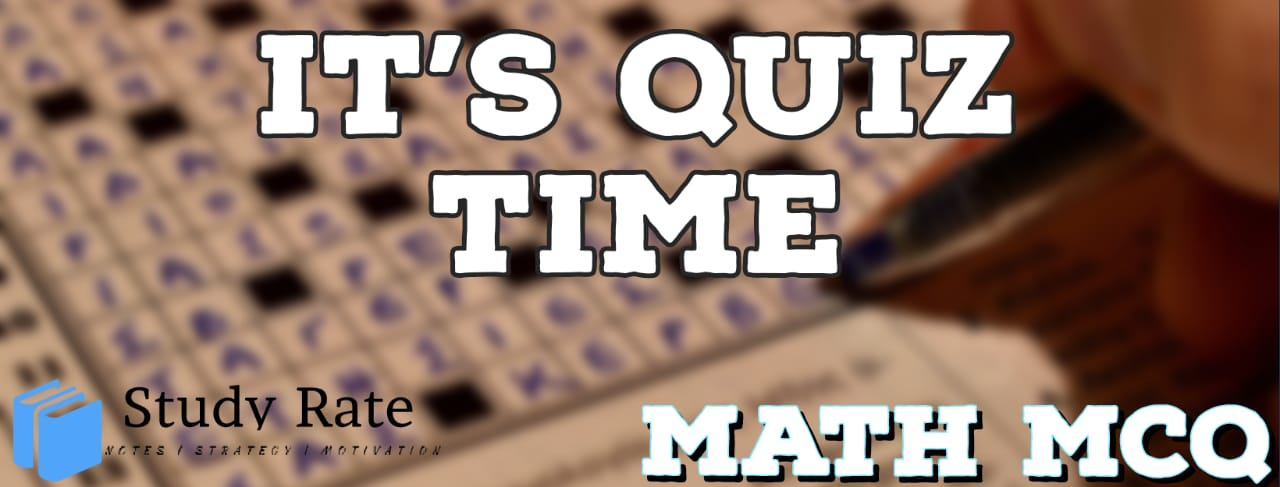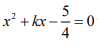## CBSE Class 10 Maths Chapter 4 –Quadratic Equations MCQ Quiz

Free PDF Download of CBSE Class 10 Maths Chapter 4 Quadratic Equations Multiple Choice Questions with Answers. MCQ Questions for Class 10 Maths with Answers was Prepared Based on Latest Exam Pattern. Students can solve Class 10 Maths Quadratic Equations MCQs with Answers to know their preparation level

Quadratic Equations Class 10 Online Quiz of MCQ’s

1. The roots of the quadratic equationare

2. Equationhas _____ real roots

3. What is the degree of a quadratic equation?

4. Find the sum of the roots of the equation5. If 1/2 is a root of the equationthen the value of k is

6. A natural number, when increased by 12, equals 160 times its reciprocal. Find the number.

7. The product of two successive integral multiples of 5 is 300. Then the numbers are:

8.  The value of9. A takes 6 days less than B to finish a piece of work. If both A and B together can finish the work in 4 days, find the time taken by B to finish the work.

10. If a train travelled 5 km/hr faster, it would take one hour less to travel 210 km. The speed of the train is :